Darcy-Weisbach Equation Calculators Enter value, select unit and click on calculate. Result will be displayed.

Calculate Acceleration Of Gravity
Calculate Pipe Diameter
Calculate Friction Factor
Calculate Pipe Length
Calculate Flow Velocity

 Web www.calculatoredge.com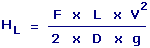HL = Head Loss F = Friction Factor V = Flow Velocity L = Pipe Length D = Pipe Diameter g = Acceleration Of Gravity
 Enter your values: Friction Factor: Pipe Length: Foot Meter Attometer Centimeter Dekameter Decimeter Exameter Femtometer Hectometer Inch Kilometer Megameter Micrometer Mile Millimeter Nanometer Petameter Picometer Yard Pipe Diameter : Foot Meter Attometer Centimeter Dekameter Decimeter Exameter Femtometer Hectometer Inch Kilometer Megameter Micrometer Mile Millimeter Nanometer Petameter Picometer Yard Flow Velocity: Foot / Second Meter / Second Centimeter / Hour Centimeter / Second Foot / Day Inch / Hour Inch / Second Kilometer / Second Knot Meter / Day Mile / Hour Mile / Second Millimeter / Second Yard / Second Acceleration Of Gravity: Foot / Second^2 Meter / Second^2 Centimeter / Second^2 Inch / Second^2 Kilometer / Second^2 Mile / Second^2 Millimeter / Second^2 Yard / Second^2 Result: Head Loss: Foot

Calculate Acceleration Of Gravity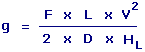g = Acceleration Of Gravity F = Friction Factor V = Flow Velocity L = Pipe Length D = Pipe Diameter HL = Head Loss
 Enter your values: Friction Factor: Head Loss: Foot Meter Attometer Centimeter Dekameter Decimeter Exameter Femtometer Hectometer Inch Kilometer Megameter Micrometer Mile Millimeter Nanometer Petameter Picometer Yard Pipe Length: Foot Meter Attometer Centimeter Dekameter Decimeter Exameter Femtometer Hectometer Inch Kilometer Megameter Micrometer Mile Millimeter Nanometer Petameter Picometer Yard Pipe Diameter : Foot Meter Attometer Centimeter Dekameter Decimeter Exameter Femtometer Hectometer Inch Kilometer Megameter Micrometer Mile Millimeter Nanometer Petameter Picometer Yard Flow Velocity: Foot / Second Meter / Second Centimeter / Hour Centimeter / Second Foot / Day Inch / Hour Inch / Second Kilometer / Second Knot Meter / Day Mile / Hour Mile / Second Millimeter / Second Yard / Second Result: Acceleration Of Gravity: Foot/Second2

Calculate Pipe Diameter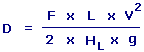D = Pipe Diameter F = Friction Factor V = Flow Velocity L = Pipe Length g = Acceleration Of Gravity HL = Head Loss
 Enter your values: Friction Factor: Head Loss: Foot Meter Attometer Centimeter Dekameter Decimeter Exameter Femtometer Hectometer Inch Kilometer Megameter Micrometer Mile Millimeter Nanometer Petameter Picometer Yard Pipe Length: Foot Meter Attometer Centimeter Dekameter Decimeter Exameter Femtometer Hectometer Inch Kilometer Megameter Micrometer Mile Millimeter Nanometer Petameter Picometer Yard Flow Velocity: Foot / Second Meter / Second Centimeter / Hour Centimeter / Second Foot / Day Inch / Hour Inch / Second Kilometer / Second Knot Meter / Day Mile / Hour Mile / Second Millimeter / Second Yard / Second Acceleration Of Gravity: Foot / Second^2 Meter / Second^2 Centimeter / Second^2 Inch / Second^2 Kilometer / Second^2 Mile / Second^2 Millimeter / Second^2 Yard / Second^2 Result: Pipe Diameter: Foot

Calculate Friction Factor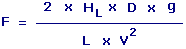F = Friction Factor D = Pipe Diameter HL = Head Loss g = Acceleration Of Gravity L = Pipe Length V = Flow Velocity
 Enter your values: Head Loss: Foot Meter Attometer Centimeter Dekameter Decimeter Exameter Femtometer Hectometer Inch Kilometer Megameter Micrometer Mile Millimeter Nanometer Petameter Picometer Yard Pipe Length: Foot Meter Attometer Centimeter Dekameter Decimeter Exameter Femtometer Hectometer Inch Kilometer Megameter Micrometer Mile Millimeter Nanometer Petameter Picometer Yard Pipe Diameter : Foot Meter Attometer Centimeter Dekameter Decimeter Exameter Femtometer Hectometer Inch Kilometer Megameter Micrometer Mile Millimeter Nanometer Petameter Picometer Yard Flow Velocity: Foot / Second Meter / Second Centimeter / Hour Centimeter / Second Foot / Day Inch / Hour Inch / Second Kilometer / Second Knot Meter / Day Mile / Hour Mile / Second Millimeter / Second Yard / Second Acceleration Of Gravity: Foot / Second^2 Meter / Second^2 Centimeter / Second^2 Inch / Second^2 Kilometer / Second^2 Mile / Second^2 Millimeter / Second^2 Yard / Second^2 Result: Friction Factor:

Calculate Pipe Length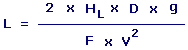L = Pipe Length D = Pipe Diameter HL = Head Loss g = Acceleration Of Gravity F = Friction Factor V = Flow Velocity
 Enter your values: Friction Factor: Head Loss: Foot Meter Attometer Centimeter Dekameter Decimeter Exameter Femtometer Hectometer Inch Kilometer Megameter Micrometer Mile Millimeter Nanometer Petameter Picometer Yard Pipe Diameter: Foot Meter Attometer Centimeter Dekameter Decimeter Exameter Femtometer Hectometer Inch Kilometer Megameter Micrometer Mile Millimeter Nanometer Petameter Picometer Yard Flow Velocity: Foot / Second Meter / Second Centimeter / Hour Centimeter / Second Foot / Day Inch / Hour Inch / Second Kilometer / Second Knot Meter / Day Mile / Hour Mile / Second Millimeter / Second Yard / Second Acceleration Of Gravity: Foot / Second^2 Meter / Second^2 Centimeter / Second^2 Inch / Second^2 Kilometer / Second^2 Mile / Second^2 Millimeter / Second^2 Yard / Second^2 Result: Pipe Length: Foot

Calculate Flow Velocity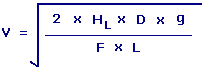V = Flow Velocity D = Pipe Diameter HL = Head Loss g = Acceleration Of Gravity F = Friction Factor L = Pipe Length
 Enter your values: Friction Factor: Head Loss: Foot Meter Attometer Centimeter Dekameter Decimeter Exameter Femtometer Hectometer Inch Kilometer Megameter Micrometer Mile Millimeter Nanometer Petameter Picometer Yard Pipe Length: Foot Meter Attometer Centimeter Dekameter Decimeter Exameter Femtometer Hectometer Inch Kilometer Megameter Micrometer Mile Millimeter Nanometer Petameter Picometer Yard Pipe Diameter : Foot Meter Attometer Centimeter Dekameter Decimeter Exameter Femtometer Hectometer Inch Kilometer Megameter Micrometer Mile Millimeter Nanometer Petameter Picometer Yard Acceleration Of Gravity: Foot / Second^2 Meter / Second^2 Centimeter / Second^2 Inch / Second^2 Kilometer / Second^2 Mile / Second^2 Millimeter / Second^2 Yard / Second^2 Result: Flow Velocity: Foot/SecondLINKSDISCLAIMERCONTACT US